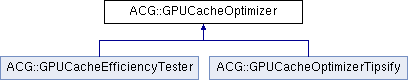Developer Documentation
ACG::GPUCacheOptimizer Class Referenceabstract

`#include <ACG/Scenegraph/MeshNodeT.hh>`

Inheritance diagram for ACG::GPUCacheOptimizer:## Classes

struct  Opt_Tris

struct  Opt_Vertex

## Public Member Functions

GPUCacheOptimizer (unsigned int NumTris, unsigned int NumVerts, unsigned int IndexSize, const void *pIndices)
constructor More...

const unsigned int * GetTriangleMap () const
Retrieves the map from dst triangle to src triangle. how to remap: for each triangle t in DstTriBuffer DstTriBuffer[t] = SrcTriBuffer[TriangleMap[t]]. More...

void WriteIndexBuffer (unsigned int DstIndexSize, void *pDst)
Applies the remapping on the initial pIndices constructor's param and stores the result in the given destination buffer. More...

unsigned int ComputeNumberOfVertexTransformations (unsigned int VertexCacheSize=16)
Returns the total number of vertex transforms performed with a certain VertexCache.

float ComputeACMR (unsigned int VertexCacheSize=16)
Measures the efficiency use of the vertex cache. ACMR: Average Cache Miss Ratio. More...

float ComputeATVR (unsigned int VertexCacheSize=16)
Measures the efficiency use of the vertex cache. ATVR: Average Transform to Vertex Ratio similar to ACMR, but easier to interpret the optimal value is 1.0 given a mesh w/o duplicate vertices. More...

## Static Public Member Functions

static void OptimizeVertices (unsigned int NumTris, unsigned int NumVerts, unsigned int IndexSize, const void *pIndices, unsigned int *pVertMap)
Reorders vertex buffer to minimize memory address jumps. More...

static void RemapVertices (unsigned int NumTris, unsigned int NumVerts, const unsigned int *pVertMap, unsigned int IndexSize, void *pInOutIndices, unsigned int VertexStride, void *pInOutVertices)
Applies the remap table of OptimizeVertices to a vertex and index buffer. More...

## Protected Member Functions

unsigned int GetIndex (unsigned int i) const

virtual void MakeAbstract ()=0

## Static Protected Member Functions

static unsigned int GetIndex (unsigned int i, unsigned int IndexSize, const void *pIB)

static void SetIndex (unsigned int i, unsigned int val, unsigned int IndexSize, void *pIB)

## Protected Attributes

unsigned int m_NumVerts

unsigned int m_NumTris

unsigned int * m_pTriMap

## Private Member Functions

GPUCacheOptimizer (const GPUCacheOptimizer &)

GPUCacheOptimizeroperator= (const GPUCacheOptimizer &)

## Private Attributes

unsigned int m_IndexSize

const void * m_pIndices

unsigned int m_NumTransformations

## Detailed Description

This class optimizes a triangle list for efficient vertex cache usage.

Definition at line 72 of file GPUCacheOptimizer.hh.

## Constructor & Destructor Documentation

 ACG::GPUCacheOptimizer::GPUCacheOptimizer ( unsigned int NumTris, unsigned int NumVerts, unsigned int IndexSize, const void * pIndices )

constructor

The constructor needs a mesh on which this node will work.

Parameters
 NumTris number of triangles NumVerts number of vertices IndexSize size in bytes of one index: 1, 2, 4 supported pIndices [in] index buffer

Definition at line 65 of file GPUCacheOptimizer.cc.

## Member Function Documentation

 float ACG::GPUCacheOptimizer::ComputeACMR ( unsigned int VertexCacheSize = `16` )

Measures the efficiency use of the vertex cache. ACMR: Average Cache Miss Ratio.

Returns
ratio: # vertex transformations / # tris

Definition at line 265 of file GPUCacheOptimizer.cc.

 float ACG::GPUCacheOptimizer::ComputeATVR ( unsigned int VertexCacheSize = `16` )

Measures the efficiency use of the vertex cache. ATVR: Average Transform to Vertex Ratio similar to ACMR, but easier to interpret the optimal value is 1.0 given a mesh w/o duplicate vertices.

Returns
ratio: # vertex transformations / # verts

Definition at line 273 of file GPUCacheOptimizer.cc.

 const unsigned int* ACG::GPUCacheOptimizer::GetTriangleMap ( ) const
inline

Retrieves the map from dst triangle to src triangle. how to remap: for each triangle t in DstTriBuffer DstTriBuffer[t] = SrcTriBuffer[TriangleMap[t]].

you can also use WriteIndexBuffer() to get the result

Definition at line 100 of file GPUCacheOptimizer.hh.

 void ACG::GPUCacheOptimizer::OptimizeVertices ( unsigned int NumTris, unsigned int NumVerts, unsigned int IndexSize, const void * pIndices, unsigned int * pVertMap )
static

Reorders vertex buffer to minimize memory address jumps.

for best performace, use AFTER triangle remapping see description on RemapVertices() on how to apply this map

Parameters
 pIndices [in] index buffer pVertMap [out] vertex remap, allocate NumVerts unsigned ints before calling dst vertex index = pVertMap[src vertex index] NOTE: if a vertex is not referenced by any triangle, the value 0xFFFFFFFF will be stored as the destination index! NumTris Number of triangles NumVerts Number of vertices IndexSize Size of the index

Definition at line 189 of file GPUCacheOptimizer.cc.

 void ACG::GPUCacheOptimizer::RemapVertices ( unsigned int NumTris, unsigned int NumVerts, const unsigned int * pVertMap, unsigned int IndexSize, void * pInOutIndices, unsigned int VertexStride, void * pInOutVertices )
static

Applies the remap table of OptimizeVertices to a vertex and index buffer.

Pseudo code for manual remapping

for each index i in IndexBuffer:
IndexBuffer[i] = VertMap[IndexBuffer[i]]
TmpBuf = VertexBuffer
for each vertex v in TmpBuf
if (VertMap[v] != 0xFFFFFFFF)
VertexBuffer[VertMap[v]] = TmpBuf[v]
Parameters
 NumVerts Number of vertices pVertMap vertex remap, result from OptimizeVertices() (input) IndexSize size in bytes of one index: 1, 2, 4 supported pInOutIndices (triangle list) index buffer, remapped after call (input/output) VertexStride size in bytes of one vertex pInOutVertices vertex buffer, remapped after call (input/output) NumTris Number of triangles

Definition at line 149 of file GPUCacheOptimizer.cc.

 void ACG::GPUCacheOptimizer::WriteIndexBuffer ( unsigned int DstIndexSize, void * pDst )

Applies the remapping on the initial pIndices constructor's param and stores the result in the given destination buffer.

Parameters
 DstIndexSize size in bytes of one index in the dst buffer pDst [out] index buffer to store the result may also be the same memory as pIndices of constructor's call NOTE: make sure pIndices is not modified/deleted between constructor's and this call

Definition at line 116 of file GPUCacheOptimizer.cc.

## Member Data Documentation

 unsigned int* ACG::GPUCacheOptimizer::m_pTriMap
protected

TriMap[new tri index] = old tri index allocated in base class, computed in child class

Definition at line 200 of file GPUCacheOptimizer.hh.

The documentation for this class was generated from the following files: